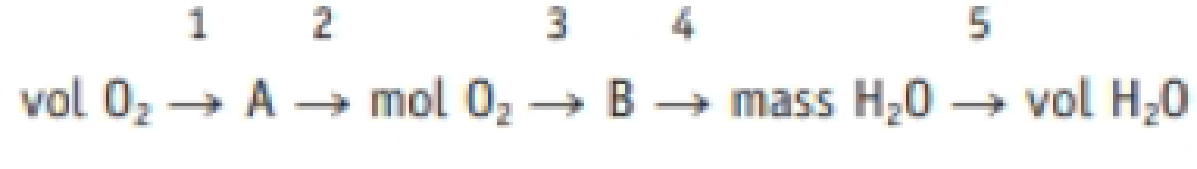Chapter 4.9, Problem 4.1ACP

Chapter
Section
Textbook Problem

Identify the factor labeled 4 in the strategy map (a) Density H2O(ℓ), 1.00 g/mL (b) Density of O2(ℓ), 1.14 g/mL (c) molar mass of O2, 32.0 g/mol (d) molar mass of H2O, 18.0 g/mol below. Labels 1 through 5 are the factors used in each step of the calculation, and A is the mass of O2 and B is the amount of H2O.Interpretation Introduction

Interpretation:

The factor labeled 4 in the given strategy map has to be identified.

Concept introduction:

• The relation between the number of moles and mass of the substance is ,

Numberofmole=MassingramMolarmass

Massingramofthesubstance=Numberofmole×Molarmass

• The molar mass of an element or compound is the mass in grams of 1 mole of that substance, and it is expressed in the unit of grams per mole (g/mol).
• Concentrationofsubstance=Amountof substancevolumeofthesubstance
• Amountof substance=Concentrationofsubstance×volumeofthesubstance
Explanation

Reason for the correct option:

The given strategy map is,

VolO21A2molO23B4MassofH2O5VolH2O

Here A is the mass of O2 and B is the amount of H2O.

The relation between the number of moles and mass of the substance is ,

Numberofmole=MassingramMolarmass

Massingramofthesu

Still sussing out bartleby?

Check out a sample textbook solution.

See a sample solution

The Solution to Your Study Problems

Bartleby provides explanations to thousands of textbook problems written by our experts, many with advanced degrees!

Get Started